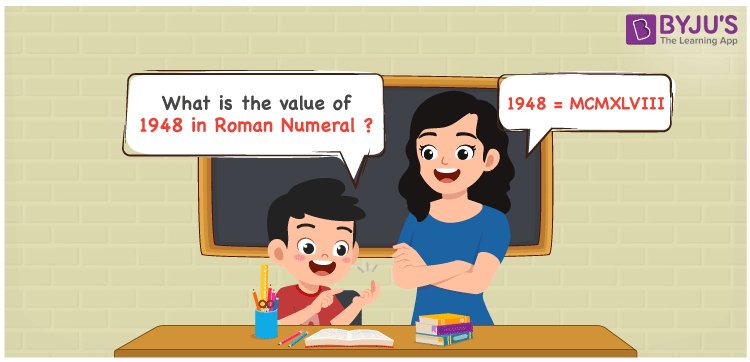# 1948 in Roman Numerals

1948 in Roman Numerals is MCMXLVIII. Master the Roman numerals article in order to get an in-depth proficiency about the conversion procedure. The steps used to expand the number and the respective roman numerals used can be memorized well in this article. Hence, 1948 can be written as MCMXLVIII in roman numerals.

 Number Roman Numeral 1948 MCMXLVIII## How to Write 1948 in Roman Numerals?

This section focuses to help students know how 1948 can be expanded based on the place value and written in Roman numerals.

1948 = 1000 + (1000 – 100) + (50 – 10) + 5 + 1 + 1 + 1

1948 = M + (M – C) + (L – X) + V + I + I + I

1948 = MCMXLVIII

## Video Lesson on Roman Numerals## Frequently Asked Questions on 1948 in Roman Numerals

Q1

### What is the value of 1948 in Roman Numerals?

In 1948,
Thousands = 1000 = M
Hundreds = 900 = CM
Tens = 40 = XL
Ones = 8 = VIII
So the number = 1000 + 900 + 40 + 8 = MCMXLVIII
Q2

### Determine 2000 – 52.

We know that
2000 in Roman Numerals = MM
52 in Roman Numerals = LII
As 2000 – 52 = 1948
So 2000 – 52 is 1948 which is written as MCMXLVIII.
Q3

### Write 1948 in Roman Numerals.

1948 can be written in Roman Numerals as MCMXLVIII by breaking the numbers according to its place value.